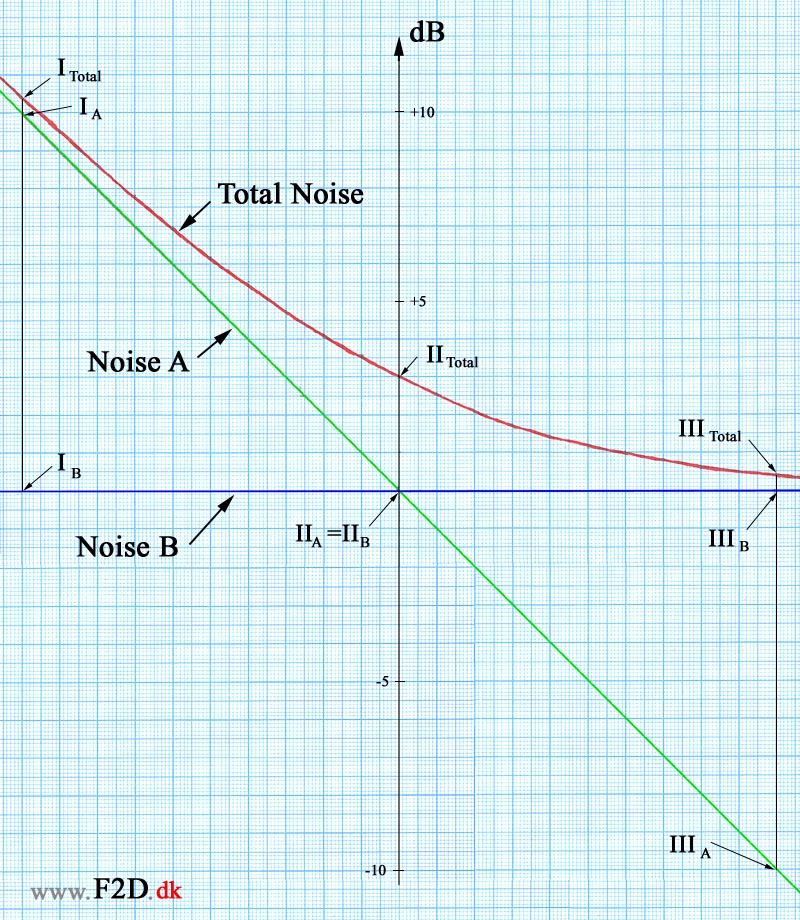Back to F2D.dk

F2D Noise Reduction
Noise Calculations
Last update: 21/10 2010

 Combining noise from two sources: It is a little tricky to make calculations on noise. The sound pressure level is measured in dB on a logarithmic scale. If a noise is a combination of noise from two sources the noise from these sources must be added as logarithmic values. In the diagram on this page a constant noise ("Noise B" - the green horizontal line) is combined with a noise ("Noise A") that change from 10 dB above the constant noise to 10 dB below this noise (the sloping green line). The combination of the two noises is the "Total Noise" (the reed curve). The diagram can be used to see how noise from two sources can be combined. Here is tree examples on different noise combinations: In the first situation (I) the noise from the first source (IA) is 10 dB higher than the noise from the second source (IB). The resulting noise (ITotal) from these two sources will be 0.4 dB higher than the noise from the first noise source. This situation is similar to a situation where the exhaust noise is 10 dB higher than the "non-exhaust" noise. The influence of the non-exhaust noise on the total noise is low and the total noise is very close to the noise level from the exhaust. In the next situation (II) the noise level from the two sources (IIA and IIB) is the same. In this situation the total noise (IITotal) will be 3 dB higher than the noise level of the two sources. In the last situation (III) the noise level from the first source (IIIA) is 10 dB lower than the noise from the second noise source (IIIB). This is similar to the first situation (I) and the total noise level is again 0.4 dB higher than the source with the highest level. This situation is similar to a situation where exhaust noise have been reduced to a level much lower than the non-exhaust noise. The level of the total noise can hardly be reduced by reducing the exhaust noise even more. If the exhaust noise was completely distinguish in this situation the total noise level would only be reduced by 0.4 dB down to the non-exhaust level.The Super Silencer Combined Noise Source & Distance CalculatorOnline calculator Sound pressure levelWikipedia Overall noise attenuation by distance(Point Source) Noise attenuation by distancen(Point Source) Noise level at immision point(Multiple Point Sources) Sound Wave Equations Formulas Calculator Loudness, Wikipedia NR-ratingThis diagram can be used to describe the effect of more effective silencers for F2D. You need to know the total noise level, the level of the non-exhaust noise and how much a new silencer will reduce the exhaust noise. The total noise can be measured with a noise level meter and the effectiveness of a silencer can be measured with a noise generator. The problem is to estimate the level of the non-exhaust noise. An attempt to estimate the non-exhaust noise with a high effective silencer can be found under the experiment with the Super Silencer. This graph was used to analyze the results from the experiments with the Super Silencer F2D.dk         < 1 2 3 4 5 6 7 8 9 10 11 12 13 14 15 16 >